# AP Chemistry : Limiting Reagent

## Example Questions

← Previous 1

### Example Question #1 : Limiting Reagent

Consider the following balanced reaction:

2Ca(s) + O2(g) → 2CaO

Consider the reaction above.  If 60g of Ca is placed in a reaction chamber with 32g of O2 which will be the limiting reactant?

There will not be a limiting reactant

O2 because there is less of it

Ca because there are 1.5 moles of Ca and only 1 mole of O2

Ca because there will need to be 2 moles if Ca for every mole of O2 as defined by the balanced equation.

O2 because it is a diatomic molecule

Ca because there will need to be 2 moles if Ca for every mole of O2 as defined by the balanced equation.

Explanation:

When considering Limiting Reactant problems the most important aspect to consider is the molar ratio of the reactants.  Here the balanced formula tells us that for every 2 moles of Ca there must be 1 mole of O2 to create the product.  The amounts given by the problem are the actual amounts we are given and can be compared to the molar ratio to determine the limiting reactant.  Since there is 1 mole of O2, in any situation in which there are less than 2 moles of Ca, Ca is the limting reactant.  Conversely if there were 2 moles of Ca, any situation in which there less than 1 mole of O2 would be a situation in which O2 is the limiting reactant.

### Example Question #2 : Limiting Reagent

Given the reaction

H2SO4 (aq) + 2 LiOH (aq) → Li2SO4 (aq) + 2 H2O (l)

If you have 100g of H2SO4 and 65g of LiOH. What is  your limiting reactant?

Both H2SO4 and LiOH limit the reaction equally

H2SO4

H2O

Li2SO4

LiOH

H2SO4

Explanation:

H2SO4 molecular weight is 98. This gives 1.02moles. Since only 1 mole is needed per reaction it allows the reaction to go through 1.02 times.

LiOH molecular weight is 24. This gives 2.71 moles. Every reaction requires 2 moles so the reaction can go through 1.35 times.

Based on this, H2SO4 is the limiting reactant.

### Example Question #1 : Limiting Reagent

What determines the amount of product formed in an irreversible reaction?

The equilbrium state

The amount of limiting reactant present

The rate of the reverse reaction

The volume of the solution

The amount of limiting reactant present

Explanation:

In irreversible reactions, the reaction proceeds in one direction only and these reactions usually go to completion. Since the forward reaction is the only one that occurs, the amount of product formed is only based on the reactants present, namely, the amount of limiting reactant.

### Example Question #4 : Limiting Reagent

Consider the following reaction: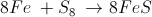If we begin with 293 g Fe and 17.2 g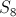, how many grams of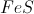will we create?

47.0 g

55.8 g

47.1 g

87.91 g

256.48 g

47.1 g

Explanation:

First, calculate the theoretical yield as if we had 293 g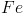and excess: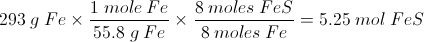Then calculate the theoretical yield as if we had 17.2 g: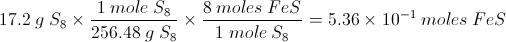The limiting reagent isas the theoretical yield calculation is lower. Now all there is to do is convert molesinto grams: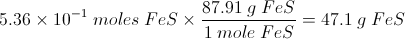### Example Question #5 : Limiting Reagent

Consider the following reaction: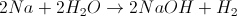If you have 36 grams of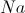and 53 mL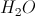, will you have any remaining sodium and/or water? How much of each?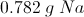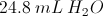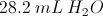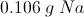There will be no remaining water or sodiumExplanation:

First, let us consider the 36 grams of solid sodium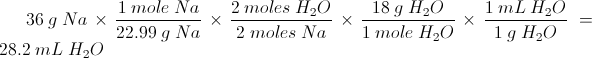We now know that sodium is the limiting reagent as it uses only 28.2 mL of water and we have 53 mL of water. We will have no remaining sodium.

We will now calculate the remaining volume of water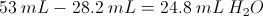### Example Question #6 : Limiting Reagent

Magnesium will combine with oxygen gas to form magnesium oxide according to the balanced equation below.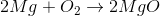60 grams of magnesium metal and 30 grams of oxygen gas are allowed to react. If the reaction runs to completion, which reactant will be depleted first?

Both will deplete at the same time.

Oxygen gas

Magnesium

Oxygen gas

Explanation:

The reactant that will be depleted first is called the limiting reagent. We can determine the limiting reagent by calculating how much oxygen gas is necessary to use up all 60 grams of magnesium.

We start by converting the magnesium to moles.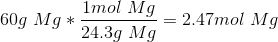We then compare the molar ratio of magnesium to oxygen gas. Since we need 1 mol of oxygen gas for every 2 moles of magnesium, we only need 1.24 moles of oxygen gas to fully react the magnesium.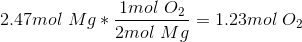Multiplying by the molar mass, we determine that we need 39.51 grams of oxygen gas. Remeber that the oxygen is diatomic in its gaseous state.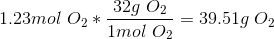Since we only have 30 grams in the givevn question, we conclude that oxygen gas will be depleted first if the reaction runs to completion.

### Example Question #7 : Limiting Reagent

Consider the following balanced reaction.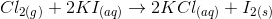If the reaction starts with 8 moles of chlorine gas and 10 moles of potassium iodide, how many moles of the excess reagent will be left over after the reaction has run to completion?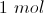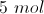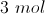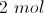Explanation:

When reading the balanced reaction, you should notice that it requires 1 mol of chlorine gas and 2 moles of potassium iodide in order to react and create the products. This ratio is seen in the coefficients of the reagents.

Using this ratio, we can calculate the mole of chlorine needed to react with 10 moles of potassium iodide.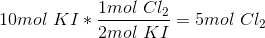5 moles of chlorine gas will be used to react all of the potassium iodide. Since we started with 8 moles of chlorine gas, this means that there are 3 moles of chlorine gas left over after the reaction has run to completion.

### Example Question #8 : Limiting Reagent

Consider the following balanced reaction.If you start with 100 grams of chlorine gas and 500 grams of potassium iodide, how many grams of the excess reagent will remain after the reaction has run to completion?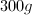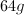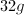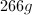Explanation:

Since we are given masses in this problem, it is a little trickier to compare the amounts of both reagents to one another. We can use stoichiometry to determine how much of one reagent is needed in order to use up all of the other reagent. We can determine how much potassium iodide is necessary to use up all of the chlorine gas by using the following series of calculations.

Convert both compounds to moles.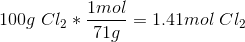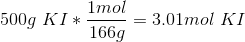Then, use the ratio in the chemical reaction to determine the moles of potassium iodide needed to react all of the chlorine.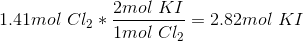Only 2.82 moles of poatssium iodide are needed. Since we started with 3.01 moles of potassium iodide, 0.19 moles will be left over. Now, we need to convert this remainsing amount to grams.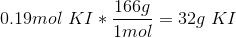### Example Question #1 : Limiting Reagent

The formation of ammonia is given by the following equation: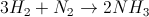If you start the reaction with 12g of hydrogen gas and 64g of nitrogen gas, which will be the limiting reagent?

We must know the system pressure in order to solve

Hydrogen

Nitrogen

Both will be used up at the same time

We must know the equilibrium constant in order to solve

Hydrogen

Explanation:

When finding the limiting reactant, it is a good idea to take one of the reactants and solve for how much of the other will be needed to fully react it. For example, let us calculate how much nitrogen gas we would need in order to use up all 12g of hydrogen gas. Using the equation to find moles, we find that we have 6mol of hydrogen gas.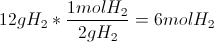Using the molar ratio of 1:3 from the chemical equation, we conclude that we only need 2mol of nitrogen gas to use up all of the hydrogen gas.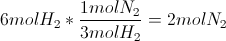Multiplying 2mol of nitrogen gas by its molar mass (28 grams per mol) gives us 56g of nitrogen gas needed to react all of the hydrogen.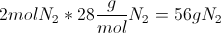Since we started with 64g of nitrogen gas, we know that we have more than enough and that hydrogen will be used up first. Hydrogen gas is the limiting reactant.

### Example Question #10 : Limiting Reagent

Consider the reaction of potassium carbonate with calcium nitrate to form potassium nitrate and calcium carbonate: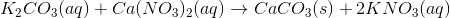Suppose 50ml of a 0.250M potassium carbonate solution was mixed with 100ml of a 0.175M calcium nitrate solution. What is the maximum amount of calcium carbonate that could be obtained?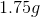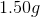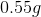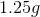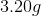Explanation:

First, we must determine how many moles of each reactant begin the reaction by multiplying the molarity by the volume. Don't forget to convert volume to liters!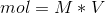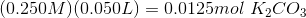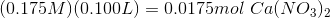Next, use the reaction coefficients (i.e. the stoichiometry) to determine how many moles of calcium carbonate could be formed from each of the reactants. In this case, there is a 1:1 molar ratio between both reactants and calcium carbonate.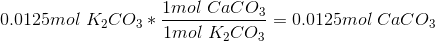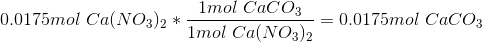Thus, 0.0125 moles of potassium carbonate could form 0.0125 moles of calcium carbonate, while 0.0175 moles of calcium nitrate could form 0.0175 moles of calcium carbonate. The maximum amount of product is going to be determined by the limiting reactant, i.e. the reactant that provides the least amount of product. In this case, the limiting reactant is potassium carbonate, and the maximum yield of calcium carbonate is 0.0125mol.

For the final step convert this value to grams: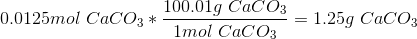← Previous 1